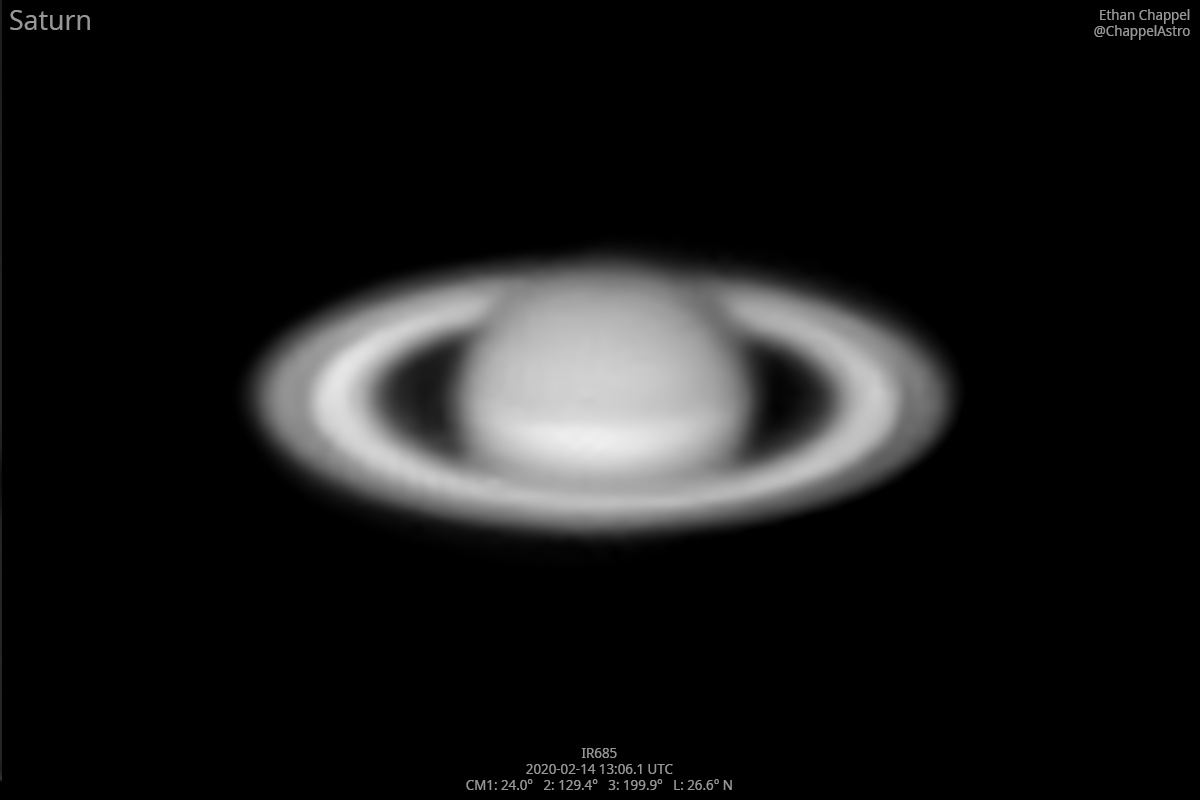# Saturn 2020-02-14 13:06 UTC

CM1: 24.00°

CM2: 129.40°

CM3: 199.90°

CLat: +26.60°

Description

Saturn was almost invisible to the naked eye by the time I finished with Jupiter. It took a few attempts to find it in the telescope. The Sun had almost risen by the time I finished the near-infrared image.

Equipment

Celestron C8 EdgeHD

ZWO ASI290MM

Astro-Physics Advanced Convertible Barlow

Logs
```FireCapture v2.6  Settings
------------------------------------
Observer=Ethan Chappel
Camera=ZWO ASI290MM
Filter=IR
Profile=Saturn
Diameter=15.20"
Magnitude=0.61
CMI=24.8° CMIII=200.7°  (during mid of capture)
FocalLength=2100mm (F/7)
Resolution=0.28"
Filename=2020-02-14-1307_5-EC-IR-Sat.ser
Date=2020_02_14
Start=13_06_19.283
Mid=13_07_34.291
End=13_08_49.300
Start(UT)=13_06_19.283
Mid(UT)=13_07_34.291
End(UT)=13_08_49.300
Duration=150.017s
Date_format=yyyy_MM_dd
Time_format=HH_mm_ss
LT=UT -6h
Frames captured=7498
File type=SER
Binning=no
Bit depth=8bit
Debayer=no
ROI=528x298
ROI(Offset)=0x0
FPS (avg.)=49
Shutter=20.00ms
Gain=260 (43%)
Brightness=1 (off)
SoftwareGain=10 (off)
HighSpeed=off
AutoExposure=off
Gamma=50
USBTraffic=40 (off)
FPS=100 (off)
AutoGain=off
AutoHisto=75 (off)
Histogramm(min)=88
Histogramm(max)=160
Histogramm=62%
Noise(avg.deviation)=7.21
AutoAlign=false
PreFilter=none
Limit=150 Seconds
Sensor temperature=9.8°C
FireCapture v2.6  Settings
------------------------------------
Observer=Ethan Chappel
Camera=ZWO ASI290MM
Filter=IR
Profile=Saturn
Diameter=15.20"
Magnitude=0.61
CMI=23.2° CMIII=199.1°  (during mid of capture)
FocalLength=2150mm (F/7)
Resolution=0.28"
Filename=2020-02-14-1304_7-EC-IR-Sat.ser
Date=2020_02_14
Start=13_03_30.130
Mid=13_04_45.133
End=13_06_00.136
Start(UT)=13_03_30.130
Mid(UT)=13_04_45.133
End(UT)=13_06_00.136
Duration=150.006s
Date_format=yyyy_MM_dd
Time_format=HH_mm_ss
LT=UT -6h
Frames captured=7497
File type=SER
Binning=no
Bit depth=8bit
Debayer=no
ROI=528x298
ROI(Offset)=0x0
FPS (avg.)=49
Shutter=20.00ms
Gain=260 (43%)
Brightness=1 (off)
SoftwareGain=10 (off)
HighSpeed=off
AutoExposure=off
Gamma=50
USBTraffic=40 (off)
FPS=100 (off)
AutoGain=off
AutoHisto=75 (off)
Histogramm(min)=65
Histogramm(max)=132
Histogramm=51%
Noise(avg.deviation)=6.31
AutoAlign=false
PreFilter=none
Limit=150 Seconds
Sensor temperature=9.8°C
```